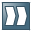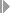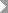Show TOC

###Creating a Unit Cost Estimate for a Sales Order Item#### Prerequisites

You have specified the costing method Unit costing in Customizing for Product Cost by Sales Order in the requirements class. If you haven't entered a default costing method, you can choose the costing method directly in the sales order.

#### Procedure

1. ChooseAccountingControllingProduct Cost ControllingCost Object ControllingProduct Cost by Sales OrderCost EstimateSales OrderChange.

The screen Change Sales Order: Initial Screen appears.

2. Enter the order and choose Enter.

3. Select an item and chooseExtrasCosting.

Choose the costing method Unit costing.

The dialog box Copy Cost Estimate appears.

4. Enter costing variant PCO4 (unit cost estimate for sales order item).

The lot size is taken from the order quantity in the sales document.

If you entered a cost estimate for an inquiry, this cost estimate is proposed when you create a cost estimate for the quotation.

If you created a cost estimate for the quotation, this cost estimate is proposed when you create a sales order.

If you want to copy a base object cost estimate, specify the desired base planning object.

5. Choose Continue.

The screen Create Unit Cost Estimate: List screen 1 appears.

6. Enter the costing items and choose Enter.

7. If you want to see the overhead before saving the cost estimate, chooseFunctionsCalculate Overhead. The system calculates the overhead using the costing sheet specified in the requirements class and possibly the overhead key in the costing sheet, and assigns the overhead to costing items of item category G.

8. If you want to use the sales order cost estimate to calculate the standard price of the valuated sales order stock, chooseFunctionsMarkingSet.

9. Save the cost estimate.

If you have not yet calculated the overhead and have entered a costing sheet and (optionally) an overhead key in Customizing in the requirements class, the system calculates the overhead when you save the cost estimate, and assigns the overhead to costing items of item category G.

#### Result

The unit cost estimate is executed. The overhead is calculated according to the costing sheet in the requirements class.

Save the sales document.

If you are using a valuated sales order stock and the cost estimate has the status VO (marked), the value calculated in the cost estimate is written to the stock segment of the sales order stock as the standard price if:

• The customer exit material valuation sales order materials was not used

• No goods receipt has taken placeNote

After the first goods receipt, the standard price of materials in the sales order or project inventory can only be changed manually. If new cost estimates are created after the first goods receipt, you might not be able to tell how the price used for valuation was calculated.

End of the note.
##### Updating the Unit Cost Estimate

If the activity prices used for valuation of the activity types have changed, or if the prices used for valuation of input materials have changed since the unit cost estimate was created, you can revaluate the items in the unit cost estimate with the current values according to the settings in the valuation variant by going into the processing mode of the unit cost estimate. You select the items that you want to revaluate, and chooseFunctionsRevaluate.